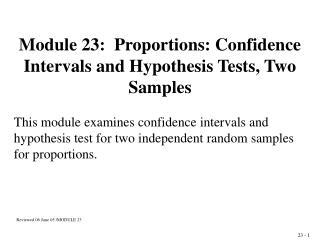DownloadDownload PresentationModule 23: Proportions: Confidence Intervals and Hypothesis Tests, Two Samples

# Module 23: Proportions: Confidence Intervals and Hypothesis Tests, Two Samples

Télécharger la présentation## Module 23: Proportions: Confidence Intervals and Hypothesis Tests, Two Samples

- - - - - - - - - - - - - - - - - - - - - - - - - - - E N D - - - - - - - - - - - - - - - - - - - - - - - - - - -
##### Presentation Transcript

1. Module 23: Proportions: Confidence Intervals and Hypothesis Tests, Two Samples This module examines confidence intervals and hypothesis test for two independent random samples for proportions. Reviewed 06 June 05 /MODULE 23 23 - 1

2. Proportions: Two Independent Random Samples P1 = Parameter for population one P2 = Parameter for population two x1 = Number in sample one with the characteristic x2 = Number in sample two with the characteristic n1 = Total number in sample one n2 = Total number in sample two p1 = x1 / n1, estimate for sample one p2 = x2 / n2, estimate for sample two 23 - 2

3. Hypothesis Test: Proportions from two samples H0: P1 = P2 vs. H1: P1  P2 p2 = x2/n2, p1 = x1/n1 The test is based on: 23 - 3

4. 23 - 5

5. Proportions for Two Independent samples 1. The hypothesis: H0: PM = PF vs. H1: PM PF 2. The assumptions: Independent random samples 3. The -level:  = 0.05 4. The test statistic: 5. The rejection region: Reject if z not between 1.96 23 - 6

6. 6. The test result: 7. The conclusion: Accept H0 : PM = PF , since z is between ±1.96 23 - 7

7. Confidence Interval for PM - PF  = 0.05 23 - 8

8. 23 - 9

9. Example: Two Vaccines In testing two new vaccines, for one group 137 of 200 persons became infected. For the second group, 98 of 150 became ill. Test the hypothesis that the two vaccines are equally effective. 1. The hypothesis: H0: P1 = P2 vs. H1: P1 P2 2. The assumptions: Independent random samples 3. The -level : = 0.05 4. The test statistic: 23 - 10

10. 5. The rejection region: Reject H0: P1= P2, if z is not between ±1.96 6. The result: 7. The conclusion: Accept H0: P1 = P2 , since z is between ±1.96 23 - 11

11. 23 - 13

12. Hypothesis Test Hypothesis test for proportion of Asthma or Wheezy Bronchitis in Social Class I vs. Social Class II nI = 191 nII = 1,173 pI = 0.047 pII = 0.078 1. The hypothesis: H0: PI = PII vs. H1: PI PII 2. The assumptions: Independent Random Samples Binomial Data 3. The -level : = 0.05 4. The test statistic: 23 - 14

13. 5. The rejection region: Reject H0: PI = PII , if z is not between ±1.96 6. The result: 7. The conclusion: Accept H0: PI = PII , since z is between ± 1.96 23 - 15

14. Confidence Interval for PI - PII nI = 191 nII = 1,173 pI = 0.047 pII = 0.078 23 - 16

15. 23 - 17